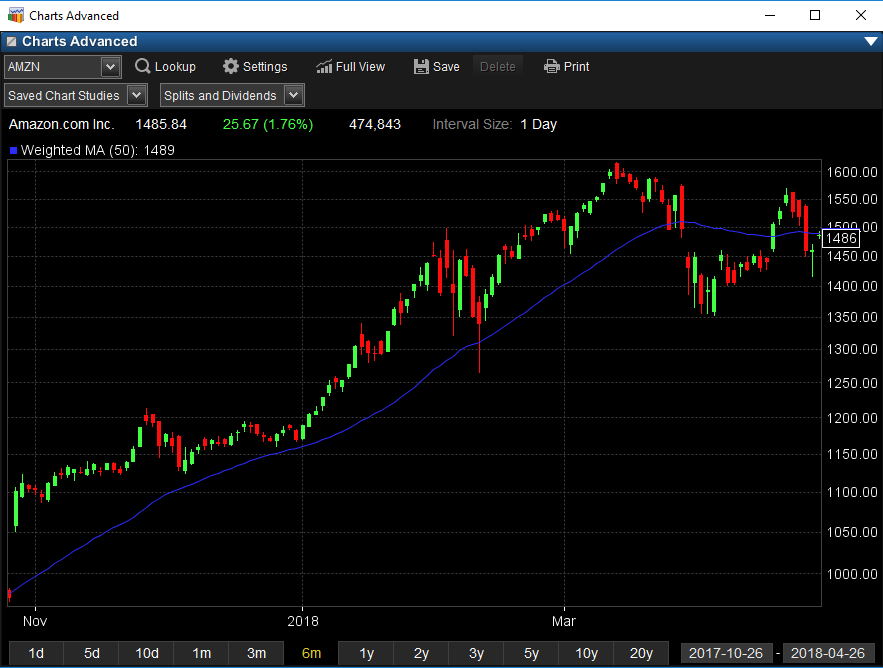Simple Moving Average

A simple, or arithmetic, moving average that is calculated by adding the closing price of the security for a number of time periods and then dividing this total by the number of time periods. Short-term averages respond quickly to changes in the price of the underlying, while long-term averages are slow to react.Exponential Moving Average

A type of moving average that is similar to a simple moving average, except that more weight is given to the latest data. The exponential moving average is also known as "exponentially weighted moving average".Weighted Moving Average

Weighted moving averages assign a heavier weighting to more current data points since they are more relevant than data points in the distant past.Wilder's Moving Average

Wilder's Moving Average was developed by Welles Wilder. The average factors the price, period and feedback from its former value, to fulfill its final calculation.